# Ramsey properties of Cayley graphs

 Importance: High ✭✭✭
 Author(s): Alon, Noga
 Subject: Graph Theory » Algebraic Graph Theory
 Keywords: Cayley graph Ramsey number
 Posted by: mdevos on: June 10th, 2007
Conjecture   There exists a fixed constantso that every abelian grouphas a subset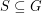withso that the Cayley graphhas no clique or independent set of size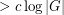.

The classic bounds from Ramsey theory show that everyvertex graph must have either a clique or an independent set of sizeand further random graphs almost surely have this property (using different values of). The above conjecture asserts that every group has a Cayley graph with similar behavior.

Improving upon some earlier results of Agarwal et. al. [AAAS], Green [G] proved that there exists a constantso that whenever a setis chosen at random, and we form the graph with vertex set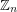and two vertices,joined if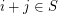, then this graph almost surely has both maximum clique size and maximum independent size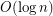. The reader should note that such graphs are not generally Cayley graphs - although the definition is similar.

As a word of caution, Green [G] also shows that a randomly chosen subset of the group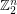almost surely has both max. clique and max. independent set of sizewhere.

## Bibliography

[AAAS] P. K. Agarwal, N. Alon, B. Aronov, S. Suri, Can visibility graphs be represented compactly? Discrete Comput. Geom. 12 (1994), no. 3, 347--365. MathSciNet

*[C] Problem BCC14.6 from the BCC Problem List (edited by Peter Cameron)

[G] B. Green, Counting sets with small sumset, and the clique number of random Cayley graphs, Combinatorica 25 (2005), no. 3, 307--326. MathSciNet

* indicates original appearance(s) of problem.